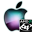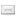ATOMS : Particle Swarm Optimization Toolbox details

# Particle Swarm Optimization Toolbox

The PSO on Scilab
Details
Version
0.4
A more recent valid version with binaries for Scilab 5.3 exists: 0.6
Author
Sébastien SALMON
Owner Organization
M3M - UTBM
Maintainers
sebastien salmon
Allan CORNET
Michael BAUDIN
Category
Creation Date
June 24, 2011
Source created on
Scilab 5.3.x
Binaries available on
Scilab 5.3.x:Windows 64-bitWindows 32-bitLinux 64-bitLinux 32-bitmacOS
Install command
`--> atomsInstall("PSO")`
Report a bugDescription
```            Purpose
-------

is based on a population of points at first stochastically
deployed on a search field.
Each member of this particle swarm could be a solution of the optimization
problem.
This swarm flies in the search field (of D dimensions) and each member
of it is attracted by its personal best solution and by the best solution
of its neighbours. Each particle has a memory storing all data relating to its
flight (location, speed and its personal best solution).
It can also inform its neighbours, i.e. communicate its speed and position.
This ability is known as socialisation. For each iteration, the objective
function is evaluated for every member of the swarm.
Then the leader of the whole swarm can be determined: it is the particle
with the best personal solution. The process leads at the end to the best
global solution.

This direct search method does not require any knowledge
of the objective function derivatives.

The PSO is a meta-heuristic optimization process created by Kennedy and Eberhart
in 1995.
Three PSO are implanted in this toolbox :
* the "Inertia Weight Model" by Shi & Eberhart in 1998,
* the "BSG-Starcraft" by the author.

Sébastien Salmon is a mecatronics research engeneer and a PhD. student at the
M3M - UTBM.
He uses the PSO for actuator optimization and inverse parameter identification.

BSG-Starcraft).
you find some bugs ;) .

Bibliography
------------

* Kennedy, J. and Eberhart, R. C. (1995). Particle swarm optimization.  Proc.
IEEE Int'l. Conf. on Neural Networks, IV, 1942–1948.  Piscataway, NJ: IEEE
Service Center.
* Shi, Y. and Eberhart, R. C. (1998a). Parameter selection in particle swarm
optimization.  In Evolutionary Programming VII: Proc. EP98, New York:
Springer-Verlag, pp. 591-600.
* Shi, Y. and Eberhart, R. C. (1998b).  A modified particle swarm optimizer.
Proceedings of the IEEE International Conference on Evolutionary Computation,
69-73. Piscataway, NJ: IEEE Press.

```Files (2)Source code archive
```
```OS-independent binary for Scilab 5.3.x
```Binary version
Automatically generated by the ATOMS compilation chain

```News (0)Comments (4)Comment from Rishi Pillai -- July 27, 2011, 10:12:44 AM
```Hi,

I wish to use PSO for data fitting to a non-linear equation.

I keep getting the error message that the size of the output function is wrong.The
condition used to print this error message would always be true in my case.I have 7
parameters (size of x) in my model but it would be always less than the size of my costf.

Here is my code,

// ******************************************************************** //
// ******************************************************************** //

xdel(winsid())
clear all

Ts=1673;
R=8.314;
fu=0.173; //not used here
inc=0.0436; //not used here
temp = 1273;
stress_s3 = 8;
E = -0.0902*temp.^2+50.794*temp+202416; //not used here
nu= 0.34; //not used here
mub= E./(2*(1+nu)); //not used here

// 1. Experimental data

x_exp_s3 = [0.0001 640 1150 1700 11634]';

y_exp_s3 = [0.0001 0.2 0.5 1 38]';

//

// Get the soze of exp data

rws = size(x_exp_s3,"r");
tlast = x_exp_s3(rws);

//

// Interpolation

xp1 = [0.0001:2500:tlast]';
xp_s3 = [xp1;tlast];
yp_s3=interp1(x_exp_s3,y_exp_s3,xp_s3,'spline');
plot(xp_s3,yp_s3,'b-',x_exp_s3,y_exp_s3,'ro');
runningPlot=2;

function f = myDifferences ( x )
// Returns the difference between the simulated
// equation and the experimental data.

theta1 = x(1)
theta2 = x(2)
theta3 = x(3)
theta4 = x(4)
A = x(5)
sig0 = x(6)
Q = x(7)

//P1 =  max(1e-10,theta1);
//P2 =  max(1e-10,theta2);
//P3 =  max(1e-10,theta3);
//P4 =  max(1e-10,theta4);
//P5 =  max(1e-10,A);
//P6 =  max(1,sig0);
//P7 =  min(7e5,Q);

y_calc = theta1.*(1-exp(-theta2.*xp_s3)) + ...
A.*sinh(stress_s3./sig0).*exp(-Q./(R.*temp)).*xp_s3 + ...
theta3.*(exp(theta4.*xp_s3)-1)
y_col = y_calc;
y_min=100*y_col;

save('yout_all.dat',y_col);
save('yout_strain_1.dat',y_min);

if runningPlot == 1 then
figure(1)
plot(xp_s3,yp_s3,'cya-',xp_s3,y_min,'r-')
drawnow;
else
end

diffmat = y_min - yp_s3;
// Make a column vector
f = diffmat./yp_s3;

endfunction

// ******************************************************************** //
// ******************************************************************** //
// End Optimisation Function

// Modified Minimization function

function val = L_Squares ( x )
// Computes the sum of squares of the differences.
f = myDifferences ( x )
val = sum(f.^2,"c")
endfunction

// ******************************************************************** //

// Optim Function

//function [f, g, ind] = modelCost (x, ind)
//  f = L_Squares ( x )
//  g = derivative ( L_Squares , x )
//endfunction

// ******************************************************************** //

// ******************************************************************** //
// ******************************************************************** //
// 3. Optimize with optim

//theta1 = 1e-10;
//theta2 = 0.000001;
//theta3 = 0.005;
//theta4 = 0.0025;
//A = 0.000001;
//sig0 = 10;
//Q = 1e5;
//
//x0 = [theta1;theta2;theta3;theta4;A;sig0;Q];
//xsc= [1e-2;1e-2;1e-2;1e-2];
//binf = [1e-15;1e-10;0.0001;0.001;1e-10;1;1e4];
//bsup = [1;300;1;0.1;10;30;5e5];
//
//[fopt,xopt,gopt]=optim(modelCost,x0,'b',binf,bsup,"qn",'ar',...
//                    300,300,1e-200,1e-200,imp=2);
//
// ******************************************************************** //
// Differential Evolution

//exec('DiffEvol.sci')

Xlow = [1e-10;1e-10;1e-10;1e-10;1e-15;1;1e4];
Xhigh = [10;10;10;10;1;100;7e5];
//
//VTR=0.01;
//Dim =7;
//Npop = 10*Dim;
//maxiter= Npop*Dim;
//Crossover= 0.5;
//Meth=2;
//select Meth
//  case 1 then strategy=1; stepsize= 1;
//  case 2 then strategy=3; stepsize= [1,0.6];
//end
//
//refresh=5;

//[x,fopt,nfeval] = DiffEvol(modelCost,VTR,Dim,Xlow,Xhigh,0,Npop,...
//                                 maxiter,stepsize,Crossover,...
//                                 strategy,refresh)
// ******************************************************************** //
// ******************************************************************** //

// Particle Swarm Optimization

bounds = [Xlow,Xhigh];
speed_max=0.1*Xhigh;
speed_min=0.1*Xlow;
speed=[speed_min,speed_max];
speedf=2;

verbose=1;

// ******************************************************************** ////
******************************************************************** //
```Comment from Rishi Pillai -- July 27, 2011, 10:15:35 AM
```Hi,

I wish to use PSO for data fitting to a non-linear equation.

I keep getting the error message that the size of the output function is wrong.The
condition used to print this error message would always be true in my case.I have 7
parameters (size of x) in my model but it would be always less than the size of my costf.

Here is my code,

// ******************************************************************** //
// ******************************************************************** //

xdel(winsid())
clear all

Ts=1673;
R=8.314;
fu=0.173; //not used here
inc=0.0436; //not used here
temp = 1273;
stress_s3 = 8;
E = -0.0902*temp.^2+50.794*temp+202416; //not used here
nu= 0.34; //not used here
mub= E./(2*(1+nu)); //not used here

// 1. Experimental data

x_exp_s3 = [0.0001 640 1150 1700 11634]';

y_exp_s3 = [0.0001 0.2 0.5 1 38]';

//

// Get the soze of exp data

rws = size(x_exp_s3,"r");
tlast = x_exp_s3(rws);

//

// Interpolation

xp1 = [0.0001:2500:tlast]';
xp_s3 = [xp1;tlast];
yp_s3=interp1(x_exp_s3,y_exp_s3,xp_s3,'spline');
plot(xp_s3,yp_s3,'b-',x_exp_s3,y_exp_s3,'ro');
runningPlot=2;

function f = myDifferences ( x )
// Returns the difference between the simulated
// equation and the experimental data.

theta1 = x(1)
theta2 = x(2)
theta3 = x(3)
theta4 = x(4)
A = x(5)
sig0 = x(6)
Q = x(7)

//P1 =  max(1e-10,theta1);
//P2 =  max(1e-10,theta2);
//P3 =  max(1e-10,theta3);
//P4 =  max(1e-10,theta4);
//P5 =  max(1e-10,A);
//P6 =  max(1,sig0);
//P7 =  min(7e5,Q);

y_calc = theta1.*(1-exp(-theta2.*xp_s3)) + ...
A.*sinh(stress_s3./sig0).*exp(-Q./(R.*temp)).*xp_s3 + ...
theta3.*(exp(theta4.*xp_s3)-1)
y_col = y_calc;
y_min=100*y_col;

save('yout_all.dat',y_col);
save('yout_strain_1.dat',y_min);

if runningPlot == 1 then
figure(1)
plot(xp_s3,yp_s3,'cya-',xp_s3,y_min,'r-')
drawnow;
else
end

diffmat = y_min - yp_s3;
// Make a column vector
f = diffmat./yp_s3;

endfunction

// ******************************************************************** //
// ******************************************************************** //
// End Optimisation Function

// Modified Minimization function

function val = L_Squares ( x )
// Computes the sum of squares of the differences.
f = myDifferences ( x )
val = sum(f.^2,"c")
endfunction

// ******************************************************************** //

// Optim Function

//function [f, g, ind] = modelCost (x, ind)
//  f = L_Squares ( x )
//  g = derivative ( L_Squares , x )
//endfunction

// ******************************************************************** //

// ******************************************************************** //
// ******************************************************************** //
// 3. Optimize with optim

//theta1 = 1e-10;
//theta2 = 0.000001;
//theta3 = 0.005;
//theta4 = 0.0025;
//A = 0.000001;
//sig0 = 10;
//Q = 1e5;
//
//x0 = [theta1;theta2;theta3;theta4;A;sig0;Q];
//xsc= [1e-2;1e-2;1e-2;1e-2];
//binf = [1e-15;1e-10;0.0001;0.001;1e-10;1;1e4];
//bsup = [1;300;1;0.1;10;30;5e5];
//
//[fopt,xopt,gopt]=optim(modelCost,x0,'b',binf,bsup,"qn",'ar',...
//                    300,300,1e-200,1e-200,imp=2);
//
// ******************************************************************** //
// Differential Evolution

//exec('DiffEvol.sci')

Xlow = [1e-10;1e-10;1e-10;1e-10;1e-15;1;1e4];
Xhigh = [10;10;10;10;1;100;7e5];
//
//VTR=0.01;
//Dim =7;
//Npop = 10*Dim;
//maxiter= Npop*Dim;
//Crossover= 0.5;
//Meth=2;
//select Meth
//  case 1 then strategy=1; stepsize= 1;
//  case 2 then strategy=3; stepsize= [1,0.6];
//end
//
//refresh=5;

//[x,fopt,nfeval] = DiffEvol(modelCost,VTR,Dim,Xlow,Xhigh,0,Npop,...
//                                 maxiter,stepsize,Crossover,...
//                                 strategy,refresh)
// ******************************************************************** //
// ******************************************************************** //

// Particle Swarm Optimization

bounds = [Xlow,Xhigh];
speed_max=0.1*Xhigh;
speed_min=0.1*Xlow;
speed=[speed_min,speed_max];
speedf=2;

verbose=1;

// ******************************************************************** ////
******************************************************************** //
```Comment from Rishi Pillai -- July 27, 2011, 10:36:41 AM
```Hi,

I wish to use PSO for data fitting to a non-linear equation.

I keep getting the error message that the size of the output function is wrong.The
condition used to print this error message would always be true in my case.I have 7
parameters (size of x) in my model but it would be always less than the size of my costf.

Here is my code,

// ******************************************************************** //
// ******************************************************************** //

xdel(winsid())
clear all

Ts=1673;
R=8.314;
fu=0.173; //not used here
inc=0.0436; //not used here
temp = 1273;
stress_s3 = 8;
E = -0.0902*temp.^2+50.794*temp+202416; //not used here
nu= 0.34; //not used here
mub= E./(2*(1+nu)); //not used here

// 1. Experimental data

x_exp_s3 = [0.0001 640 1150 1700 11634]';

y_exp_s3 = [0.0001 0.2 0.5 1 38]';

//

// Get the soze of exp data

rws = size(x_exp_s3,"r");
tlast = x_exp_s3(rws);

//

// Interpolation

xp1 = [0.0001:2500:tlast]';
xp_s3 = [xp1;tlast];
yp_s3=interp1(x_exp_s3,y_exp_s3,xp_s3,'spline');
plot(xp_s3,yp_s3,'b-',x_exp_s3,y_exp_s3,'ro');
runningPlot=2;

function f = myDifferences ( x )
// Returns the difference between the simulated
// equation and the experimental data.

theta1 = x(1)
theta2 = x(2)
theta3 = x(3)
theta4 = x(4)
A = x(5)
sig0 = x(6)
Q = x(7)

//P1 =  max(1e-10,theta1);
//P2 =  max(1e-10,theta2);
//P3 =  max(1e-10,theta3);
//P4 =  max(1e-10,theta4);
//P5 =  max(1e-10,A);
//P6 =  max(1,sig0);
//P7 =  min(7e5,Q);

y_calc = theta1.*(1-exp(-theta2.*xp_s3)) + ...
A.*sinh(stress_s3./sig0).*exp(-Q./(R.*temp)).*xp_s3 + ...
theta3.*(exp(theta4.*xp_s3)-1)
y_col = y_calc;
y_min=100*y_col;

save('yout_all.dat',y_col);
save('yout_strain_1.dat',y_min);

if runningPlot == 1 then
figure(1)
plot(xp_s3,yp_s3,'cya-',xp_s3,y_min,'r-')
drawnow;
else
end

diffmat = y_min - yp_s3;
// Make a column vector
f = diffmat./yp_s3;

endfunction

// ******************************************************************** //
// ******************************************************************** //
// End Optimisation Function

// Modified Minimization function

function val = L_Squares ( x )
// Computes the sum of squares of the differences.
f = myDifferences ( x )
val = sum(f.^2,"c")
endfunction

// ******************************************************************** //

// Optim Function

//function [f, g, ind] = modelCost (x, ind)
//  f = L_Squares ( x )
//  g = derivative ( L_Squares , x )
//endfunction

// ******************************************************************** //

// ******************************************************************** //
// ******************************************************************** //
// 3. Optimize with optim

//theta1 = 1e-10;
//theta2 = 0.000001;
//theta3 = 0.005;
//theta4 = 0.0025;
//A = 0.000001;
//sig0 = 10;
//Q = 1e5;
//
//x0 = [theta1;theta2;theta3;theta4;A;sig0;Q];
//xsc= [1e-2;1e-2;1e-2;1e-2];
//binf = [1e-15;1e-10;0.0001;0.001;1e-10;1;1e4];
//bsup = [1;300;1;0.1;10;30;5e5];
//
//[fopt,xopt,gopt]=optim(modelCost,x0,'b',binf,bsup,"qn",'ar',...
//                    300,300,1e-200,1e-200,imp=2);
//
// ******************************************************************** //
// Differential Evolution

//exec('DiffEvol.sci')

Xlow = [1e-10;1e-10;1e-10;1e-10;1e-15;1;1e4];
Xhigh = [10;10;10;10;1;100;7e5];
//
//VTR=0.01;
//Dim =7;
//Npop = 10*Dim;
//maxiter= Npop*Dim;
//Crossover= 0.5;
//Meth=2;
//select Meth
//  case 1 then strategy=1; stepsize= 1;
//  case 2 then strategy=3; stepsize= [1,0.6];
//end
//
//refresh=5;

//[x,fopt,nfeval] = DiffEvol(modelCost,VTR,Dim,Xlow,Xhigh,0,Npop,...
//                                 maxiter,stepsize,Crossover,...
//                                 strategy,refresh)
// ******************************************************************** //
// ******************************************************************** //

// Particle Swarm Optimization

bounds = [Xlow,Xhigh];
speed_max=0.1*Xhigh;
speed_min=0.1*Xlow;
speed=[speed_min,speed_max];
speedf=2;

verbose=1;

// ******************************************************************** ////
******************************************************************** //
```Comment -- September 12, 2011, 10:55:29 AM
```Hi,

This message is just to note that we discussed this topic by personal message.

Regards,

Michaël```Leave a comment
Login withEmail notifications